Mobile QR Code1. (Georgia Institute of Technology, Analog Devices Inc)

Design, analysis, stability, high bandwidth, dc–dc power supply, hysteretic current-mode control

## I. POWERING WIRELESS MICROSYSTEMS

Emerging wireless microsystems sense, process, and report information that can save money, energy, and lives . With so much functionality and so little space, however, their onboard batteries deplete easily. So to conserve energy, they idle when possible and wake only on demand, and often quickly and to high-power states. Power supplies must therefore be compact, efficient, and fast.

Switched-inductor dc–dc power supplies are popular in many applications because they can deliver over 90% of the power they draw [2-4]. When pulse-width-modulated (PWM), they normally require multiple switching cycles to respond and recover after sudden load dumps . Hysteretic converters are faster because they can slew the inductor's current uninterruptedly until the current can satisfy the load and replenish the output capacitor . Slewing the inductor this way is the fastest possible way that a switched inductor can respond to load variations.

Although already analyzed in different ways and from different perspectives in literature [7-9], stability requirements for these hysteretic power supplies remain largely algebraic, abstract, and theoretical (without experimental validation). This brief explains and demonstrates with experiments how those requirements relate to the inductor and the load dumps it supports. With this insight, a designer can more readily stabilize and identify under which operating conditions a hysteretic power supply will remain stable. To explain this, Sections 2–5 describe circuit operation, stability requirements, validating measurements, and relevant conclusions.

## II. HYSTERETIC CURRENT-MODE CONVERTER

### 1. Operation

The hysteretic current-mode buck dc–dc converter in Fig. 1 closes a feedback loop that, in keeping output $v_O$ near reference $v_R$, supplies the current that the load requires. Transconductor $G_{OSC}$ is an oscillator that ripples inductor $L_O$'s current $i_L$ across a window that comparator $CP_{OSC}$'s hysteretic thresholds set and about a level that amplifier $A_E$ dictates with $v_{ERR}$. So together, $A_E$ compares $v_O$ and $v_R$ to generate an error $v_{ERR}$ that adjusts the level about which $G_{OSC}$ oscillates $i_L$ to match and supply the current $i_{LD}$ that the load demands.

Fig. 2. Measured inductor current in continuous conduction mode (CCM).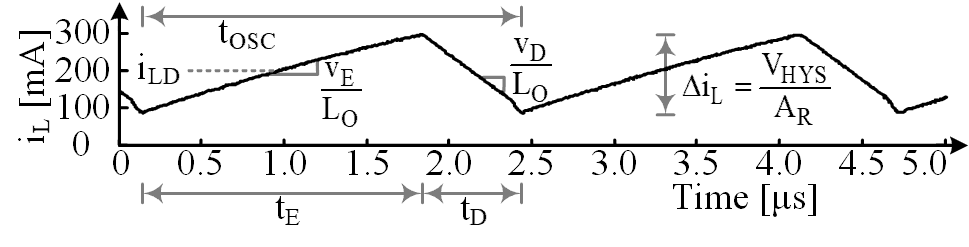The system delivers power by energizing and draining $L_O$ from the input $v_{IN}$ into $v_O$ in alternating phases of the oscillating period tOSC. When transistor $M_{IN}$ energizes $L_O$ with energizing voltage $v_E$ or $v_{IN}$ – $v_O$, $i_L$ in Fig. 2 rises across energizing period $t_E$\. $i_L$ similarly falls when switch $M_G$ drains $L_O$ with drain voltage $v_D$ or –$v_O$ across drain period $t_D$. $i_L$ ripples this way about the load level the feedback loop sets with $v_{ERR}$

When $L_O$'s and $C_{IL}$'s corner frequencies with $R_{IL}$ and RLESR are well below the oscillating frequency f$_{OSC}$, $sL_O$ and $R_{IL}$ overwhelm RLESR and 1/$sC_{IL}$ near f$_{OSC}$. So $L_O$'s voltage $v_L$ is $i_L$$sL_O, v_C is v_L/sR_{IL}$$C_{IL}$ or $i_L$$L_O/R_{IL}$$C_{IL}$, and $v_I$ is $i_L$(10$L_O$/$R_{IL}$$C_{IL}) or i_L$$A_R$ where current sense gain $A_R$ equals:

##### (1)
$A_{\mathrm{R}}=\frac{v_{\mathrm{I}}}{i_{\mathrm{L}}}=10\left(\frac{L_{\mathrm{O}}}{R_{\mathrm{IL}}C_{\mathrm{IL}}}\right)$

$v_I$ therefore rises and falls with $i_L$, and when $v_I$ reaches comparator $CP_{OSC}$'s upper threshold, $CP_{OSC}$ trips low to open $M_{IN}$ and close $M_G$, and that way, end $t_E$. The opposite happens when $v_I$ falls to $CP_{OSC}$'s lower threshold to end $t_D$. $i_L$ oscillates this way across the window that $CP_{OSC}$'s hysteresis $V_{HYS}$ sets with V$_{HYS}$/$A_R$ and about the level that $v_{ERR}$ sets with $v_{ERR}$/$A_R$. Together, $R_{IL}$, $C_{IL}$, $CP_{OSC}$, $M_{IN}$, $M_G$, and $L_O$ realize an oscillator $G_{OSC}$ that oscillates $i_L$ about $i_{LD}$.

Fig. 3. Measured inductor current in discontinuous conduction mode (DCM).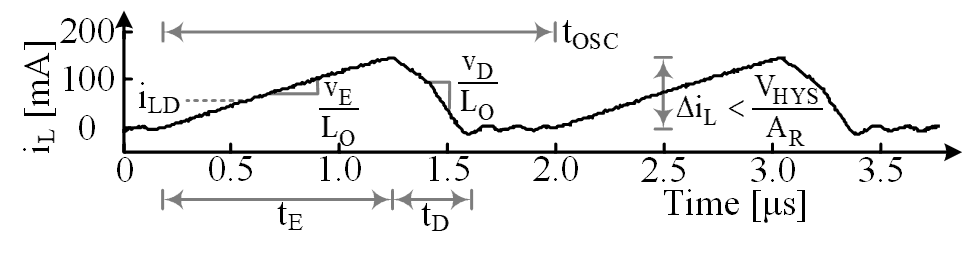Fig. 4. Voltage-mode hysteretic control for buck converters.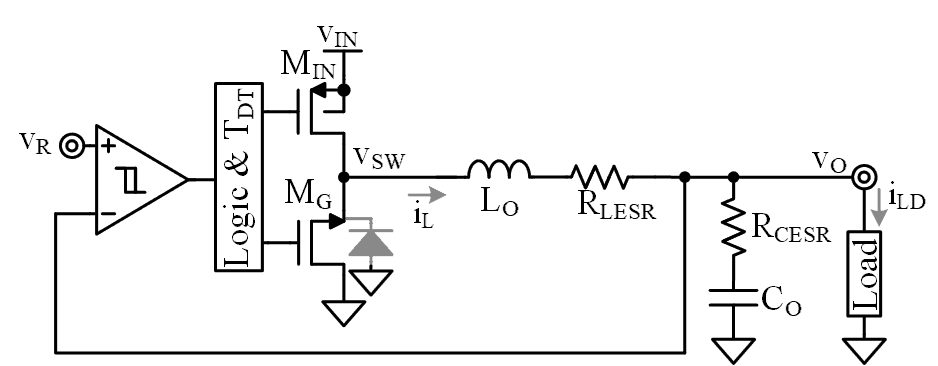When load current $i_{LD}$ falls below half of $i_L$'s ripple Δ$i_L$, $i_L$ can reverse, and that way, burn unnecessary power. Comparator $CP_{ZCS}$ keeps this from happening by sensing and opening $M_G$ when $i_L$ reaches zero. In this way, like Fig. 3 shows, $i_L$ falls to zero and remains at zero in discontinuous-conduction mode (DCM) until the next cycle. But since Δ$i_L$ is less than prescribed by V$_{HYS}$/$A_R$, $v_O$ must rise above $v_R$ to trip $CP_{OSC}$. In other words, $v_O$ rises slightly when $i_{LD}$ falls in DCM.

### 2. Variants

The voltage-mode counterpart of the hysteretic current-mode buck is the most compact hysteretic buck because $v_O$ and $v_R$ feed directly into $CP_{OSC}$ like Fig. 4 shows , without using $A_E$ or a current sensor. Unfortunately, the circuit requires a resistive $C_O$, the equivalent series resistance (ESR) of which produces higher ripple in $v_O$. Adding a current sensor without gain and feeding its output $v_I$ into $CP_{OSC}$ remove this requirement [11,12]. This way, $CP_{OSC}$ keeps $v_O$ near $v_R$ because $v_I$ is $v_O$ + $v_C$. $v_C$'s $i_L$$L_O/R_{IL}$$C_{IL}$, however, appears as an offset in $v_O$. The purpose of $A_E$ in Fig. 1 is to eliminate this offset. The state of the art adds peripheral blocks to Fig. 1 to keep the oscillating frequency constant [11-13]. These additions, however, do not alter the stability limits and effects of the hysteretic core.

Fig. 5. System-level block diagram modeling current loop as transconductance G$_{\mathrm{OSC}}$.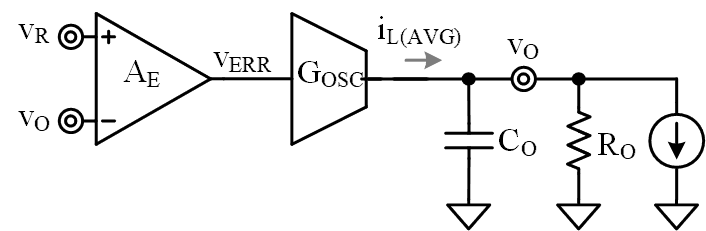Hysteretic current-mode boost and buck–boost configurations are also possible. What changes in these configurations are the voltages $v_E$ and $v_D$ that energize and drain $L_O$. $v_E$, for example, is $v_{IN}$ instead of $v_{IN}$ – $v_O$, and $v_D$ in the boost is $v_{IN}$ – $v_O$ instead of just –$v_O$ . So as long as the analysis is with respect to $v_E$ and $v_D$, the mechanics explained here apply to all configurations. The output diode or switch in boost and buck–boost topologies, however, produce the effect of an out-of-phase, right-hand-plane (RHP) zero $z_{RHP}$ that does not appear in buck converters . But as long as the unity-gain frequency $f_{0dB}$ is below $z_{RHP}$, which is a necessary requirement for these converters, all stability conditions are the same.

## III. STABILITY

For the feedback loop that controls $v_{\mathrm{O}}$ to stabilize, the loop gain $A_{\mathrm{LG}}$ must reach unity (at $f_{\mathrm{0dB}}$) with less than 180$^{\circ}$ of phase shift. Across the loop (in Fig. 5), $G_{\mathrm{OSC}}$'s bandwidth establishes one pole $p_{\mathrm{G}}$ and output capacitor $C_{\mathrm{O}}$ shunts $v_{\mathrm{O}}$ to produce another pole $p_{\mathrm{O}}$. $C_{\mathrm{O}}$'s series resistance $R_{\mathrm{CESR}}$ limits $C_{\mathrm{O}}$'s shunting current to eliminate the effects of $p_{\mathrm{O}}$ past zero $z_{\mathrm{CESR}}$. But $R_{\mathrm{CESR}}$ for low-ripple applications is so low that $z_{\mathrm{CESR}}$ is negligibly high . And $C_{\mathrm{O}}$ is so high that $p_{\mathrm{O}}$ is low and dominant. A phase margin of 45$^{\circ}$ balances speed and stability by limiting the number of damped oscillating rings to three or less . So to maintain 45$^{\circ}$ of phase margin PM, $f_{\mathrm{0dB}}$ should be at or below $p_{\mathrm{G}}$:

##### (2)
$PM=180^{o}- Tan^{- 1}\left(\frac{f_{0dB}}{p_{O}}\right)- Tan^{- 1}\left(\frac{f_{0dB}}{p_{G}}\right)$.

$p_G$ is another way to quantify $G_{OSC}$'s delay time $t_D$ . In the case of hysteretic current-mode converters, $t_D$ is the time $L_O$ requires to slew $i_L$ across a load dump Δ$i_{LD}$. Because $i_L$ climbs faster with higher $L_O$ voltage $v_L$ ($v_{SW}$ – $v_O$ in Fig. 1), $t_D$ is longer and $p_G$ is lower when energizing and drain $v_L$ voltages $v_E$ and $v_D$ are lower and Δ$i_{LD}$ is higher. And since $i_L$ requires about four time constants 4τG to reach 98% of its target, $t_D$ is roughly 4τG or τG is $t_D$/4 and $p_G$ is no less than the lowest $v_L$ and highest Δ$i_{LD}$ dictate:

##### (3)
$\mathrm{p}_{\mathrm{G}}=\frac{1}{2\pi \tau _{G}}\approx \left(\frac{1}{2\pi }\right)\left(\frac{4}{t_{D}}\right)=\left(\frac{1}{2\pi }\right)\left(\frac{4}{\Delta i_{LD}}\right)\left(\frac{v_{L}}{L_{O}}\right)$

At worst case conditions, this yields:

##### (4)
$\mathrm{p}_{\mathrm{G}(\mathrm{$M_{IN}$})}=\frac{4Min\left| v_{E},v_{D}\right| }{2\pi \Delta i_{\mathrm{LD}(\mathrm{MAX})}L_{O}}$

In practice, the application defines $v_{IN}$, $v_O$, and Δ$i_{LD}$ and volume, power-rating, and conversion-efficiency constraints limit $L_O$. So for the fastest 45° response, $C_O$ should be just high enough to define a p$_O$ that keeps $f_{0dB}$ near the minimum $p_G$ or $p_G$($M_{IN}$) that these parameters set. To quantify this, first consider that $A_{LG}$ is the gain across $A_E$ and $G_{OSC}$ into the output impedance that $R_O$ and $C_O$ in parallel establish:

##### (5)
$\mathrm{p}_{\mathrm{o}}=\frac{1}{2 \pi \mathrm{R}_{\mathrm{o}} \mathrm{C}_{\mathrm{o}}}$

##### (6)
\begin{aligned} \mathrm{A}_{\mathrm{LG}}=\left.\mathrm{A}_{\mathrm{E}} \mathrm{G}_{\mathrm{OSC}}\left(\mathrm{R}_{\mathrm{O}} \| \frac{1}{s \mathrm{C}_{\mathrm{O}}}\right)\right|_{\mathrm{p}_{0}<<{\mathrm{f}_\mathrm{0dB}} \leq \mathrm{P}_{\mathrm{G(M_{IN})}}} \\ \approx \mathrm{A}_{\mathrm{E}} \mathrm{G}_{\mathrm{OSCO}} \mathrm{R}_{\mathrm{O}}\left(\frac{\mathrm{p}_{\mathrm{o}}}{\mathrm{f}_{\mathrm{ooB}}}\right) \end{aligned}

where $G_{OSC0}$ is $G_{OSC}$'s low-frequency translation 1/$A_R$:

##### (7)
$\mathrm{G}_{\mathrm{OSC}0}=\frac{\mathrm{i}_{\mathrm{l}}}{\mathrm{v}_{\mathrm{err}}}\approx \frac{1}{\mathrm{A}_{\mathrm{R}}}$

Since $p_G$'s effect on $A_{LG}$ near $f_{0dB}$ is small and $A_{LG}$ at $f_{0dB}$ is one, $f_{0dB}$ is roughly equivalent to $A_{LG}$'s gain–bandwidth product:

##### (8)
\begin{aligned} \mathrm{f}_{0 \mathrm{dB}} \approx \mathrm{A}_{\mathrm{LG} 0} \mathrm{p}_{\mathrm{O}}=\left(\mathrm{A}_{\mathrm{E}} \mathrm{G}_{\mathrm{osco}} \mathrm{R}_{\mathrm{o}}\right) \mathrm{p}_{\mathrm{o}} \\ =\frac{\mathrm{A}_{\mathrm{E}} \mathrm{G}_{\mathrm{osc} 0} \mathrm{R}_{\mathrm{o}}}{2 \pi \mathrm{R}_{\mathrm{o}} \mathrm{C}_{\mathrm{o}}}=\left(\frac{\mathrm{A}_{\mathrm{E}}}{\mathrm{A}_{\mathrm{R}}}\right)\left(\frac{1}{2 \pi \mathrm{C}_{\mathrm{o}}}\right) \leq \mathrm{p}_{\mathrm{G(M_{IN})}} \end{aligned}

where $A_{LG0}$ is $A_{LG}$'s low-frequency translation $A_E$$G_{OSC0}$$R_O$. The Bode plot in Fig. 6 illustrates pole locations and the small-signal dynamics needed for stable conditions.

When worst-case extremes for $v_{IN}$, $v_O$, Δ$i_{LD}$, and $L_O$ in the buck of Fig. 1 are 1.1 V, 1 V, 150 mA, and 3.3 μH, $v_E$'s $v_{IN}$ – $v_O$ or 100 mV is lower than $v_D$'s $v_O$ or 1 V. So $p_G$ can be 130 kHz, and for 45° of phase margin, $f_{0dB}$ should not exceed $p_G$'s 130 kHz. $C_O$ should therefore be no less than 15 μF when $A_E$ is 12 V/V, $R_{IL}$ is 33 kΩ, and $C_{IL}$ is 1 nF, which means $A_R$ is 1 Ω.

Relative to the state of the art, the analysis in  requires a model of the system in the form of complex state-space matrices. Although  and  decompose the system into transfer functions, their expressions are still complex. So drawing intuition to understand how each component in the system affects stability is challenging.

The analysis presented here is much easier to understand and implement. Expressions are insightful and correspond directly to components in the system. The analysis does not require state-space matrices. And thanks to its segmentation, the analysis is scalable with respect to additional gain stages with poles and zeros.

## IV. PROTOTYPE

### 1. Hardware

The 0.6-$mm^2$ 0.18-μm CMOS die in Fig. 7 integrates the error amplifier $A_E$, oscillating comparator $CP_{OSC}$, dead-time logic, power transistors $M_{IN}$ and $M_G$ and their drivers, and zero-current sensing comparator $CP_{ZCS}$. The current sensor, inductor $L_O$, and output capacitor $C_O$ are off chip on the two-layer board alongside test circuits used for experiments. $L_O$ and $C_O$ measure 10.7 × 10 × 5.4 and 1.6 × 0.81 × 0.91 mm3 and incorporate 15 and 4 mΩ, respectively.

Fig. 7. Prototyped 0.18-μm CMOS die and two-layer board.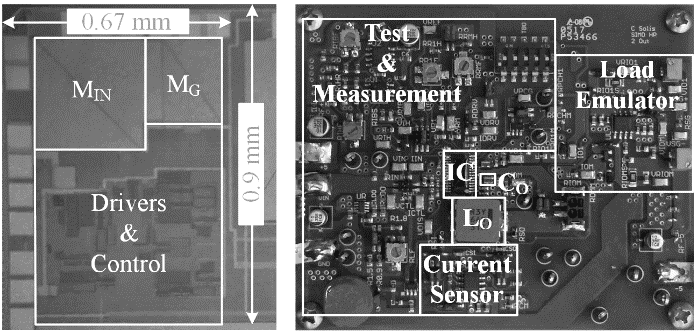Fig. 8. Measured output voltage regulation across output load and input voltage.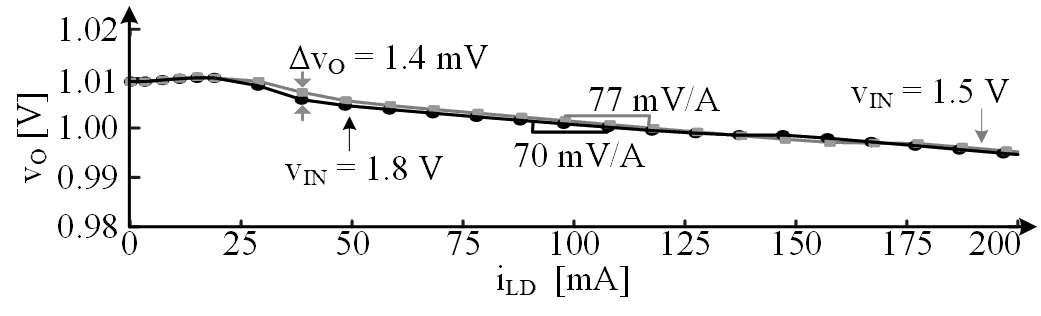Fig. 9. Measured response to 40-, 80-, and 180-mA load dumps.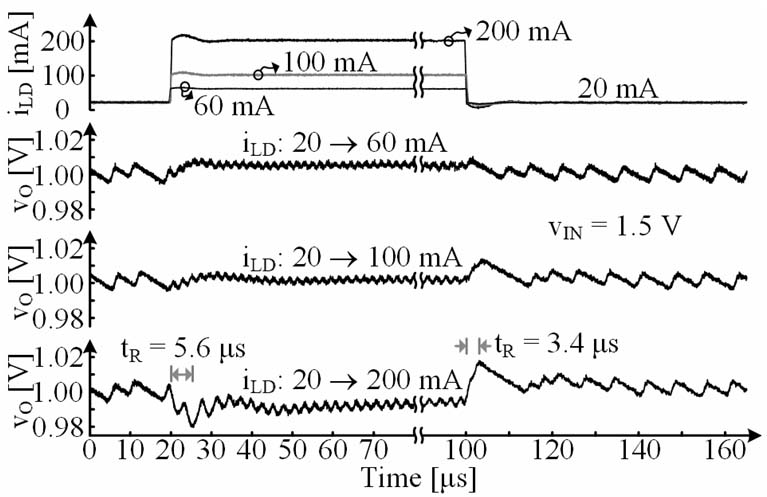Fig. 8 shows the average output voltage $v_O$ across load level $i_{LD}$ at two input voltage $v_{IN}$ operating points. $v_O$ decreases with increasing $i_{LD}$ due to its finite output resistance at a rate, i.e. load regulation, of 77 mV/A. Across the same loading, the worst-case output voltage difference $\Delta v_O$ is 1.4 mV which results in a line regulation of 4.7 mV/V.

### 2. Response

The measured output $v_O$ in Fig. 9 ripples 5 to 10 mV and responds within 5.6 μs to rising 40-, 80-, and 180-mA load dumps. Response time tR is basically how long $L_O$ requires to slew $i_L$ across these load steps. The system responds faster (within 3.4 μs) to similar falling load dumps because $L_O$'s drain voltage $v_D$ is higher at $v_O$'s 1.0 V than $L_O$'s energizing counterpart $v_E$, which is $v_{IN}$ – $v_O$ or 1.5 – 1.0 V, which is 0.5 V.

Fig. 10. Measured load-dump response when the input is 1.4 and 1.8 V.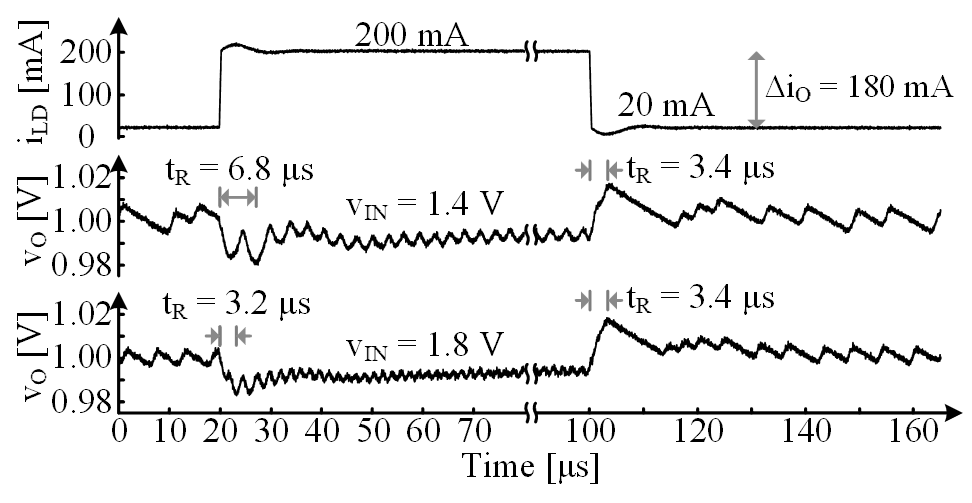Phase margin essentially quantifies the propensity of a feedback loop to oscillate when perturbed. In this case, $v_O$ in Fig. 5 is more prone to ringing when responding to rising than to falling load dumps. This is because $L_O$ requires more time to respond to rising loads. In other words, the bandwidth pole of the oscillator is lower, and as a result, closer to the unity-gain frequency $f_{0dB}$ of the loop. Similarly, the ringing worsens as the load step increases from 40 to 180 mA because $L_O$ requires more time to slew across wider load steps. Ringing also worsens as input voltage falls in Fig. 10 from 1.8 to 1.4 V for the same reason, because with a lower voltage across $L_O$, $i_L$ slews more slowly.

Using Eqs. (2-8), phase margins for 40-, 80-, and 180-mA load dumps are 75°, 62° and 40°, respectively. These calculated margins correspond well with Fig. 9. The 40-mA load dump response, for example, is closer to the over-damped response that 90° produces. The under-damped response to the 180-mA load dump rings 2–3 times before settling. This corresponds to the response that 45° produces.

### 3. Efficiency

$A_E$, $CP_{OSC}$, $CP_{ZCS}$, $M_{IN}$, $M_G$, the drivers, and dead-time logic consume quiescent, ohmic, and switching power P$_Q$, PR, and $P_{SW}$. As load current $i_{LD}$ climbs, output power $P_O$ increases linearly, whereas PR rises quadratically. Up to at least 200 mA, however, $P_O$ outpaces PR, so power-conversion efficiency ηC in Fig. 11 generally increases with $i_{LD}$. Although PR is very low below 5 mA, $P_O$ is also low, so P$_Q$ and $P_{SW}$ become large fractions of $P_O$. This is why ηC drops below 88% abruptly when $i_L$ is less than 5 mA.

## V. CONCLUSIONS

Hysteretic current-mode converters are compact, fast, and efficient, but although widely stable, not immune to instabilities. The bandwidth delay of the oscillating current loop unfortunately introduces a secondary pole that falls with lower inductor voltages and higher load-dump currents. This is why the 200-mA, 1-V, 0.18-μm CMOS buck prototyped here responds more slowly to rising than to falling load dumps and rings more with lower input voltages and higher load steps. Understanding these stability limits is critical when designing hysteretic current-mode power supplies, which given their size, speed, and efficiency benefits, are increasingly prevalent in consumer electronics.

### ACKNOWLEDGMENTS

The authors thank J.D. Morris, B. Legates, T. Bonte, and Analog Devices Inc. for their sponsorship and support.

### REFERENCES

1
Puccinelli D., Haenggi M., 2005, Wireless Sensor Networks Applications and Challenges of Ubiquitous Sensing, IEEE Circuits and Systems Magazine, Vol. 5, No. 3, pp. 19-312
Mathúna C.O., Ningning W., Kulkarni S., Roy S., Nov 2012, Review of Integrated Magnetics for Power Supply on Chip (PwrSoC), IEEE Transactions on Power Electronics, Vol. 27, No. 11, pp. 4799-48163
Hwan C., Byun S., 2016, A 82.5% Power Efficiency at 1.2 mW Buck Converter with Sleep Control, Jounral of Semiconductor Technology and Science, Vol. 16, No. 6, pp. 842-8464
Kim K.H., Kong B.S., 2005, Ultra-fast Adaptive Frequency-controlled Hysteretic Buck Converter for Portable Devices, Jounral of Semiconductor Technology and Science, Vol. 16, No. 5, pp. 615-6235
Duan X., Huang A. Q., Jul 2006, Current-Mode Variable-Frequency Control Architecture for High-Current Low-Voltage DC–DC Converters, IEEE Transactions on Power Electronics, Vol. 21, No. 4, pp. 1133-11376
Keskar N., Rincón-Mora G.A., May 2007, Designing an Accurate and Robust LC-Compliat Asynchronous DS Boost DC-DC Converter, IEEE International Symposium on Circuits and Systems, pp. 549-5527
Mattavelli P., Rossetto L., Spiazzi G., Jan 1997, Small-Signal Analysis of DC–DC Converters with Sliding Mode Control, IEEE Transactions on Power Electronics, Vol. 12, No. 1, pp. 96-1028
Mishra S.K., Ngo K.D.T., Apr 2009, Dynamic Characterization of the Synthetic Ripple Modulator in a Tightly Regulated Distributed Power Application, IEEE Transactions on Industrial Electronics, Vol. 56, No. 4, pp. 1164-11739
Sun J., Jul 2002, Small-Signal Modeling of Variable-Frequency Pulsewidth Modulators, IEEE Transactions on Aerospace and Electronic Systems, Vol. 38, No. 3, pp. 1104-110810
Hu K.Y., Lin S.M., Tsai C.H., Oct 2015, A Fixed-Frequency Quasi-V2 Hysteretic Buck Converter With PLL-Based Two-Stage Adaptive Window Control, IEEE Transactions on Circuits and Systems I: Regular Papers, Vol. 62, No. 10, pp. 2565-257311
Lee S.H., Bang J.S., Yoon K.S., Hong S.W., Shin C.S., Jung M.Y., Cho G.H., Feb 2015, A 0.518$mm^2$ quasi-current-mode hysteretic buck DC-DC converter with 3μs load transient response in 0.35μm BCDMOS, IEEE International Solid-State Circuits Conference, pp. 214-21512
Chien S.H., Hung T.H., Huang S.Y., Kuo T.H., Nov 2015, A Monolithic Capacitor-Current-Controlled Hysteretic Buck Converter With Transient-Optimized Feedback Circuit, IEEE Journal of Solid-State Circuits, Vol. 50, No. 11, pp. 2524-253213
Fayed M., Nashed A., 2017, A Current-Mode Hysteretic Buck Converter with Spur-Free Control for Variable Switching Noise Mitigation, IEEE Transactions on Power Electronics14
Rincon-Mora G. A., 2016, Power IC Design - Fifth Edition, Lulu, Raleigh15
Su F., Ki W.H., Feb 2009, Digitally Assisted Quasi-V2 Hysteretic Buck Converter with Fixed Frequency and without Using Large-ESR Capacitor, IEEE International Solid-State Circuits Conference, pp. 446-44716
Allen P.E., Holberg D. R., 2002, CMOS Analog Circuit Design, Oxford University Press, New York17
Solis C.J., Rincón-Mora G.A., Dec 2013, Stability Analysis & Design of Hysteretic Current-Mode Switched-Inductor Buck DC-DC Converters, International Conference on Electronics, Circuits and Systems, pp. 811-814## Author

Carlos Solis received the B.S. degree from the University of Puerto Rico at Mayaguez in 2010, and the M.S. and Ph.D. degree from the Georgia Institute of Technology in 2012 and 2018, all in electrical engineering.

His research interests include switching power supplies, and analog IC design.

He is currently in Analog Devices as an Analog Design Engineer.

Gabriel A. Rincón-Mora has been a Professor at Georgia Tech since 2001, Visiting Professor at National Cheng Kung University since 2011, and Design Team Leader at Texas Instruments in 1994–2003.

He is a Fellow of the National Academy of Inventors, Fellow of the Institute of Electrical and Electronics Engineers, and Fellow of the Institution of Engineering and Technology.

Recognitions include induction into Georgia Tech's Council of Outstanding Young Engineering Alumni, National Hispanic in Technology Award, Charles E. Perry Visionary Award, Three-Year Patent Award, Orgullo Hispano Award, Hispanic Heritage Award, and a State of California Commendation Certificate.

His scholarly output includes 9 books, 2 handbooks, 4 book chapters, 42 licensed patents, over 180 articles, over 26 commercial power-chip products, and over 150 lectures/keynotes/speeches.